xTrapezoidOverview

In Euclidean geometry
Euclidean geometry
Euclidean geometry is a mathematical system attributed to the Alexandrian Greek mathematician Euclid, which he described in his textbook on geometry: the Elements. Euclid's method consists in assuming a small set of intuitively appealing axioms, and deducing many other propositions from these...

, a convex
Convex set
In Euclidean space, an object is convex if for every pair of points within the object, every point on the straight line segment that joins them is also within the object...

quadrilateral
Quadrilateral
In Euclidean plane geometry, a quadrilateral is a polygon with four sides and four vertices or corners. Sometimes, the term quadrangle is used, by analogy with triangle, and sometimes tetragon for consistency with pentagon , hexagon and so on...

with one pair of parallel sides is referred to as a trapezoid in American English
American English
American English is a set of dialects of the English language used mostly in the United States. Approximately two-thirds of the world's native speakers of English live in the United States....

and as a trapezium in English outside North America. A trapezoid with vertices
Vertex (geometry)
In geometry, a vertex is a special kind of point that describes the corners or intersections of geometric shapes.-Of an angle:...

ABCD is denoted
Mathematical notation
Mathematical notation is a system of symbolic representations of mathematical objects and ideas. Mathematical notations are used in mathematics, the physical sciences, engineering, and economics...

. The parallel sides are called the bases of the trapezoid.

This article uses the term trapezoid in the sense that is current in the United States (and sometimes in some other English-speaking countries).Unanswered QuestionsWhat's special about a trapezoid?How are the diagonals of a trapezoid and its median related?Encyclopedia
In Euclidean geometry
Euclidean geometry
Euclidean geometry is a mathematical system attributed to the Alexandrian Greek mathematician Euclid, which he described in his textbook on geometry: the Elements. Euclid's method consists in assuming a small set of intuitively appealing axioms, and deducing many other propositions from these...

, a convex
Convex set
In Euclidean space, an object is convex if for every pair of points within the object, every point on the straight line segment that joins them is also within the object...

quadrilateral
Quadrilateral
In Euclidean plane geometry, a quadrilateral is a polygon with four sides and four vertices or corners. Sometimes, the term quadrangle is used, by analogy with triangle, and sometimes tetragon for consistency with pentagon , hexagon and so on...

with one pair of parallel sides is referred to as a trapezoid in American English
American English
American English is a set of dialects of the English language used mostly in the United States. Approximately two-thirds of the world's native speakers of English live in the United States....

and as a trapezium in English outside North America. A trapezoid with vertices
Vertex (geometry)
In geometry, a vertex is a special kind of point that describes the corners or intersections of geometric shapes.-Of an angle:...

ABCD is denoted
Mathematical notation
Mathematical notation is a system of symbolic representations of mathematical objects and ideas. Mathematical notations are used in mathematics, the physical sciences, engineering, and economics...

. The parallel sides are called the bases of the trapezoid.

This article uses the term trapezoid in the sense that is current in the United States (and sometimes in some other English-speaking countries). Readers in the United Kingdom and Australia should read trapezium for each use of trapezoid in the following paragraphs. In all other languages using a word derived from the Greek for this figure, the form closest to trapezium (e.g. French 'trapèze', Italian 'trapezio', German 'Trapez', Russian 'трапеция') is used.

The term trapezium has been in use in English since 1570, from Late Latin trapezium, from Greek trapezion, literally "a little table", diminutive of trapeza "table", itself from tra- "four" + peza "foot, edge". The first recorded use of the Greek word translated trapezoid (τραπεζοειδη, table-like) was by Marinus Proclus
Proclus
Proclus Lycaeus , called "The Successor" or "Diadochos" , was a Greek Neoplatonist philosopher, one of the last major Classical philosophers . He set forth one of the most elaborate and fully developed systems of Neoplatonism...

(412 to 485 AD) in his Commentary on the first book of Euclid’s Elements.

## Definition

There is also some disagreement on the allowed number of parallel sides in a trapezoid. At issue is whether parallelogram
Parallelogram
In Euclidean geometry, a parallelogram is a convex quadrilateral with two pairs of parallel sides. The opposite or facing sides of a parallelogram are of equal length and the opposite angles of a parallelogram are of equal measure...

s, which have two pairs of parallel sides, should be counted as trapezoids. Some authors define a trapezoid as a quadrilateral having exactly one pair of parallel sides, thereby excluding parallelograms. Other authors define a trapezoid as a quadrilateral with at least one pair of parallel sides, making the parallelogram a special type of trapezoid (along with the rhombus
Rhombus
In Euclidean geometry, a rhombus or rhomb is a convex quadrilateral whose four sides all have the same length. The rhombus is often called a diamond, after the diamonds suit in playing cards, or a lozenge, though the latter sometimes refers specifically to a rhombus with a 45° angle.Every...

, the rectangle
Rectangle
In Euclidean plane geometry, a rectangle is any quadrilateral with four right angles. The term "oblong" is occasionally used to refer to a non-square rectangle...

and the square
Square (geometry)
In geometry, a square is a regular quadrilateral. This means that it has four equal sides and four equal angles...

). The latter definition is consistent with its uses in higher mathematics such as calculus. The former definition would make such concepts as the trapezoidal approximation to a definite integral be ill-defined.

## Special cases

In an isosceles trapezoid
Isosceles trapezoid
In Euclidean geometry, an isosceles trapezoid is a convex quadrilateral with a line of symmetry bisecting one pair of opposite sides, making it automatically a trapezoid...

, the base angles have the same measure, and the other pair of opposite sides AD and BC also have the same length.

In a right trapezoid, two adjacent angles are right angles.

## Characterizations

A convex quadrilateral is a trapezoid if and only if
If and only if
In logic and related fields such as mathematics and philosophy, if and only if is a biconditional logical connective between statements....

it has two adjacent angle
Angle
In geometry, an angle is the figure formed by two rays sharing a common endpoint, called the vertex of the angle.Angles are usually presumed to be in a Euclidean plane with the circle taken for standard with regard to direction. In fact, an angle is frequently viewed as a measure of an circular arc...

s that are supplementary
Supplementary angles
Supplementary angles are pairs of angles that add up to 180 degrees. Thus the supplement of an angle of x degrees is an angle of degrees....

, that is, they add up 180 degree
Degree (angle)
A degree , usually denoted by ° , is a measurement of plane angle, representing 1⁄360 of a full rotation; one degree is equivalent to π/180 radians...

s.

Another necessary and sufficient condition is that the diagonal
Diagonal
A diagonal is a line joining two nonconsecutive vertices of a polygon or polyhedron. Informally, any sloping line is called diagonal. The word "diagonal" derives from the Greek διαγώνιος , from dia- and gonia ; it was used by both Strabo and Euclid to refer to a line connecting two vertices of a...

s cut each other in mutually the same ratio
Ratio
In mathematics, a ratio is a relationship between two numbers of the same kind , usually expressed as "a to b" or a:b, sometimes expressed arithmetically as a dimensionless quotient of the two which explicitly indicates how many times the first number contains the second In mathematics, a ratio is...

(this ratio is the same as that between the lengths of the parallel sides).

A convex quadrilateral is also a trapezoid if and only if the diagonals form one pair of similar opposite triangles

## Area

The area K of a simple (that is, not self-intersecting) trapezoid is given by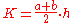where a and b are the lengths of the parallel sides, and h is the height (the perpendicular distance between these sides.) In 499 CE Aryabhata
Aryabhata
Aryabhata was the first in the line of great mathematician-astronomers from the classical age of Indian mathematics and Indian astronomy...

, a great mathematician
Mathematician
A mathematician is a person whose primary area of study is the field of mathematics. Mathematicians are concerned with quantity, structure, space, and change....

-astronomer
Astronomer
An astronomer is a scientist who studies celestial bodies such as planets, stars and galaxies.Historically, astronomy was more concerned with the classification and description of phenomena in the sky, while astrophysics attempted to explain these phenomena and the differences between them using...

from the classical age of Indian mathematics
Indian mathematics
Indian mathematics emerged in the Indian subcontinent from 1200 BCE until the end of the 18th century. In the classical period of Indian mathematics , important contributions were made by scholars like Aryabhata, Brahmagupta, and Bhaskara II. The decimal number system in use today was first...

and Indian astronomy, used this method in the Aryabhatiya
Aryabhatiya
Āryabhaṭīya or Āryabhaṭīyaṃ, a Sanskrit astronomical treatise, is the magnum opus and only extant work of the 5th century Indian mathematician, Āryabhaṭa.- Structure and style:...

(section 2.8).
This yields as a special case the well-known formula for the area of a triangle
Triangle
A triangle is one of the basic shapes of geometry: a polygon with three corners or vertices and three sides or edges which are line segments. A triangle with vertices A, B, and C is denoted ....

, by considering a triangle as a degenerate trapezoid in which one of the parallel sides has shrunk to a point.

The mid-segment of a trapezoid is the segment that joins the midpoints of the non-parallel sides. Its length m is equal to the average of the lengths of the bases of the trapezoid: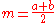It follows that the area of a trapezoid is equal to the length of this mid-segment multiplied by the height: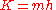In the case that the two parallel sides are different lengths (ab), the height of a trapezoid h, and hence its area A can be determined by the length of all of its sides: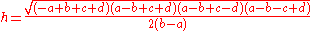where, c and d are the lengths of the other two sides, and hence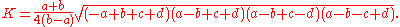When one of the parallel sides has shrunk to a point (say a = 0), this formula reduces to Heron's formula for the area of a triangle.

Another equivalent formula for the area, which more closely resembles Heron's formula is: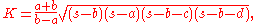where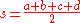is the semiperimeter
Semiperimeter
In geometry, the semiperimeter of a polygon is half its perimeter. Although it has such a simple derivation from the perimeter, the semiperimeter appears frequently enough in formulas for triangles and other figures that it is given a separate name...

of the trapezoid. (This formula is similar to Brahmagupta's formula
Brahmagupta's formula
In Euclidean geometry, Brahmagupta's formula finds the area of any quadrilateral given the lengths of the sides and some of the angles. In its most common form, it yields the area of quadrilaterals that can be inscribed in a circle.- Basic form :...

, but it differs from it, in that a trapezoid might not be cyclic
Cyclic quadrilateral
In Euclidean geometry, a cyclic quadrilateral is a quadrilateral whose vertices all lie on a single circle. This circle is called the circumcircle or circumscribed circle, and the vertices are said to be concyclic. Other names for these quadrilaterals are chordal quadrilateral and inscribed...

(inscribed in a circle). The formula is also a special case of Bretschneider's formula for a general quadrilateral
Quadrilateral
In Euclidean plane geometry, a quadrilateral is a polygon with four sides and four vertices or corners. Sometimes, the term quadrangle is used, by analogy with triangle, and sometimes tetragon for consistency with pentagon , hexagon and so on...

).

Therefore using Bretschneider's formula gives: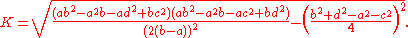The centre of area (centre of mass for a uniform lamina) lies along the line joining the mid-points of the parallel sides, at a perpendicular distance d from the longer side b given by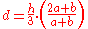## Properties

The line joining the mid-points of the parallel sides bisects the area.
If the trapezoid is divided into 4 triangles by its diagonals AC and BD (as shown on the right), intersecting at O, then the area of is equal to that of , and the product of the areas of and is equal to that of and . The ratio of the areas of each pair of adjacent triangles is the same as that between the lengths of the parallel sides.

Therefore the diagonal length is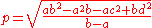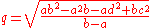Let the trapezoid have vertices A, B, C, and D in sequence and have parallel sides AB and DC. Let E be the intersection of the diagonals, and let F be on side DA and G be on side BC such that FEG is parallel to AB and CD. Then FG is the harmonic mean
Harmonic mean
In mathematics, the harmonic mean is one of several kinds of average. Typically, it is appropriate for situations when the average of rates is desired....

of AB and DC: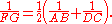If M and N are the midpoints of the diagonals, then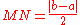where a and b are the lengths of the parallel sides.

If the angle bisectors to angles A and B intersect at P, and the angle bisectors to angles C and D intersect at Q, then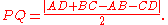The line joining the point where the nonparallel sides intersect and the intersection point of the diagonals bisect each base.

## Terminology

The the term trapezium is sometimes defined in the USA as a quadrilateral
Quadrilateral
In Euclidean plane geometry, a quadrilateral is a polygon with four sides and four vertices or corners. Sometimes, the term quadrangle is used, by analogy with triangle, and sometimes tetragon for consistency with pentagon , hexagon and so on...

with no parallel sides, though this shape is more usually called an irregular quadrilateral.. The term trapezoid was once defined as a quadrilateral without any parallel sides in Britain and elsewhere, but this does not reflect current usage. (The Oxford English Dictionary says “Often called by English writers in the 19th century".)

According to the Oxford English Dictionary
Oxford English Dictionary
The Oxford English Dictionary , published by the Oxford University Press, is the self-styled premier dictionary of the English language. Two fully bound print editions of the OED have been published under its current name, in 1928 and 1989. The first edition was published in twelve volumes , and...

, the sense of a figure with no sides parallel is the meaning for which Proclus
Proclus
Proclus Lycaeus , called "The Successor" or "Diadochos" , was a Greek Neoplatonist philosopher, one of the last major Classical philosophers . He set forth one of the most elaborate and fully developed systems of Neoplatonism...

introduced the term "trapezoid". This is retained in the French "trapézoïde", German "Trapezoid", and in other languages. A trapezium in Proclus' sense is a quadrilateral having one pair of its opposite sides parallel. This was the specific sense in England in 17th and 18th centuries, and again the prevalent one in recent use. A trapezium as any quadrilateral more general than a parallelogram is the sense of the term in Euclid
Euclid
Euclid , fl. 300 BC, also known as Euclid of Alexandria, was a Greek mathematician, often referred to as the "Father of Geometry". He was active in Alexandria during the reign of Ptolemy I...

. The sense of a trapezium as an irregular quadrilateral having no sides parallel was the sometimes used in England from c1800 to c1875, but is now obsolete. This sense is the one that is sometimes quoted in the U.S., but in practice quadrilateral is used rather than trapezium.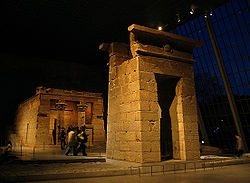## Architecture

In architecture the word is used to refer to symmetrical doors, windows, and buildings built wider at the base, tapering towards the top, in Egyptian style. If these have straight sides and sharp angular corners, their shapes are in fact isoceles trapezoids.

## See also

• Isosceles trapezoid
Isosceles trapezoid
In Euclidean geometry, an isosceles trapezoid is a convex quadrilateral with a line of symmetry bisecting one pair of opposite sides, making it automatically a trapezoid...

• Parallelogram
Parallelogram
In Euclidean geometry, a parallelogram is a convex quadrilateral with two pairs of parallel sides. The opposite or facing sides of a parallelogram are of equal length and the opposite angles of a parallelogram are of equal measure...

• Polite number
Polite number
In number theory, a polite number is a positive integer that can be written as the sum of two or more consecutive positive integers. Other positive integers are impolite....

, also known as a trapezoidal number
• Trapezoidal rule

## External links

The source of this article is wikipedia, the free encyclopedia.  The text of this article is licensed under the GFDL.